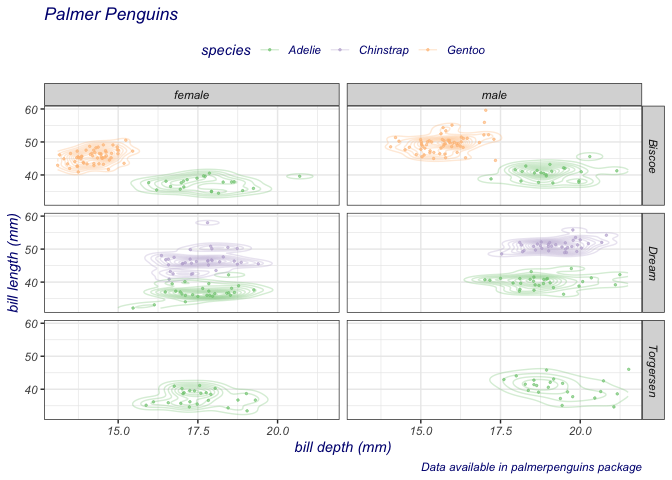## Formula interface to ggplot2

ggformula introduces a family of graphics functions, gf_point(), gf_density(), and so on, bring the formula interface to ggplot(). This captures and extends the excellent simplicity of the lattice-graphics formula interface, while providing the intuitive “add this component” capabilities of ggplot2.

## Installation

You can install ggformula from CRAN sith

install.packages("ggformula")

or from github with:

# install.packages("devtools")
devtools::install_github("ProjectMOSAIC/ggformula")

## Using ggformula

The following example illustrates a typical plot constructed with ggformula.

suppressPackageStartupMessages(library(ggformula))
data(penguins, package = "palmerpenguins")
penguins %>%
set_variable_labels(
bill_length_mm = "bill length (mm)",
bill_depth_mm = "bill depth (mm)"
) %>%
gf_jitter(bill_length_mm ~ bill_depth_mm | island ~ sex, color = ~ species,
width = 0.05, height = 0.05, size = 0.5, alpha = 0.6) %>%
gf_density2d(alpha = 0.3) %>%
gf_labs(title = "Palmer Penguins",
caption = "Data available in palmerpenguins package"
) %>%
gf_refine(scale_color_brewer(type = "qual")) %>%
gf_theme(theme_bw()) %>%
gf_theme(
legend.position = 'top',
text = element_text(colour = "navy", face = "italic")
)
#> Warning: Removed 2 rows containing non-finite values (stat_density2d).
#> Warning: Computation failed in stat_density2d():
#> missing value where TRUE/FALSE needed
#> Warning: Removed 2 rows containing missing values (geom_point).Find out more about ggformula at https://www.mosaic-web.org/ggformula/.